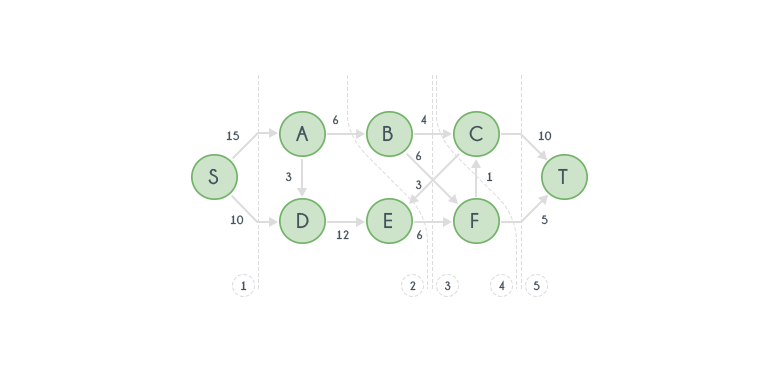Algorithms
Topics:
Min-cut
• Searching
• Sorting
• Greedy Algorithms
• Graphs
• String Algorithms
• Dynamic Programming

# Min-cut

Min-Cut of a weighted graph is defined as the minimum sum of weights of (at least one)edges that when removed from the graph divides the graph into two groups. Mechthild Stoer and Frank Wagner proposed an algorithm in 1995 to find minimum cut in an undirected weighted graphs.

The algorithm works on a method of shrinking the graph by merging the most tightly connected vertex until only one node is left in the graph and for each step performed, the weight of the merged cut is stored in a list. Minimum value in the list would be the minimum cut value of the graph.

Pseudocode for the algorithm is given below,

function: MinCutPhase(Graph G, Weights W, Vertex a):
A <- {a}
while A != V:
add tightly connected vertex to A
store cut_of_the_phase and shrink G by merging the two vertices added last

minimum = INF
function: MinCut(Graph G, Weights W, Vertex a):
while |V| > 1:
MinCutPhase(G,W,a)
if cut_of_the_phase < minimum:
minimum = cut_of_the_phase
return minimum


Vertex $a$ can be arbitrary and remains same throughout the whole algorithm. $A$ in MinCutPhase is a collection of vertices which starts with the arbitrary vertex $a$ and starts adding other vertices of graph $G$ one at a time until it is equal to $V$ which is collection of all vertices. Tightly connected vertex to $A$ implies a vertex whose edge weight to any of the vertex in $A$ is maximum. It can mathematically represented as $z \not\in A\ such\ that\ W(A,z) = max\{W(A,y) | y \not\in A\}$, $z$ will be the new tightly connected vertex. The cut_of_the_phase is the sum of edges connecting last added vertex with rest of the graph. Minimum value of $cut\_of\_the\_phase$ is the required result.

Last two vertices added to the set $A$ are merged by creating a single node for both of them and edges joining them are deleted. Edges connecting these vertices to other vertices are replaced with edges weighing sum of edges to both the vertex. Ex: Let vertices $P$ and $Q$ are the two vertices added last to the set $A$. Let there are three edges connecting $P$ and $Q$ to the set $A$, $E(X,P), W(X,P) = 10; E(X,Q), W(X,Q) = 20; E(Y,Q), W(Y,Q) = 15$. Now as they are added last, vertices $P$ and $Q$ are merged to a single node, say $R$. Now, edges connecting to $R$ will be, $E(X,R), W(X,R) = 30; E(Y,R), W(Y,R) = 15$.

For a flow network with $a$ as in the algo mentioned above, is either $S$ or $T$, will be the maximum flow of the network from $S$ to $T$. This is because, minimum cut will be the bottleneck capacity of the flow network. And always, the amount of flow from source side to sink side has to pass through these set of edges that are to be cut. Working on a paper, source should be on left and destination on right. The cut should always start from top of the graph, move to bottom of the graph(should not stop in between). The edges that are to be considered in min-cut should move from left of the cut to right of the cut. Sum of capacity of all these edges will be the min-cut which also is equal to max-flow of the network.

Working on a directed graph to calculate max flow of the graph using min-cut concept is shown in image below.Few possible cuts in the graph are shown in the graph and weights of each cut are as follows: $Cut 1: 25, Cut 2: 12, Cut 3: 16, Cut 4: 10, Cut 5: 15$. As mentioned these are only few possible cuts but considering any valid cut would not have weight less than $Cut 4$. And it is the min-cut of this graph which is also the max-flow of the graph as explained above.

Contributed by: Vinay Kumar

?